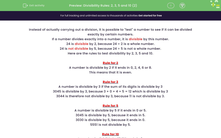# Know the Divisibility Rules for 2, 3, 5 and 10

In this worksheet, students will revise and practise the rules for divisibility by 2, 3, 5 and 10 to find the missing digits.Key stage:  KS 3

Curriculum topic:   Number

Curriculum subtopic:   Use Concepts and Vocabulary for All Numbers

Difficulty level:#### Worksheet Overview

Instead of actually carrying out a division, it is possible to test a number to see if it can be divided exactly by certain numbers.

If a number divides exactly into a number, it is divisible by this number.

24 is divisible by 2 because 24 ÷ 2 is a whole number.

24 is not divisible by 5 because 24 ÷ 5 is not a whole number.

Here are the rules to test divisibility by 2, 3, 5 and 10.

Rule for 2

A number is divisible by 2 if it ends in 0, 2, 4, 6 or 8.

This means that it is even.

Rule for 3

A number is divisible by 3 if the sum of its digits is divisible by 3

3045 is divisible by 3 because 3 + 0 + 4 + 5 = 12 which is divisible by 3

3044 is therefore not divisible by 3 because 11 is not divisible by 3.

Rule for 5

A number is divisible by 5 if it ends in 0 or 5.

3045 is divisible by 5 because it ends in 5.

3030 is divisible by 5 because it ends in 0.

5551 is not divisible by 5.

Rule for 10

A number is divisible by 10 if it ends in 0.

3140 is divisible by 10 because it ends in 0.

3145 is not divisible by 10.

Example1

What digit must replace the ? to make the number 501? divisible by 5?

? can be 0 or 5.

Example 2

What digit must replace the ? to make the number 501?2 divisible by 3?

So far, 5 + 0 + 1 + 2 = 8

This means that ? could equal 1, 4 or 7 to give a digit sum of 9, 12 or 15, which are all divisible by 3.

This seems a lot to remember, so feel free to check back to the introduction at any point by clicking on the red button at the side of the screen.

### What is EdPlace?

We're your National Curriculum aligned online education content provider helping each child succeed in English, maths and science from year 1 to GCSE. With an EdPlace account you’ll be able to track and measure progress, helping each child achieve their best. We build confidence and attainment by personalising each child’s learning at a level that suits them.

Get started••••